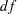## Example 26.1 Estimating Covariances and Correlations

This example shows how you can use PROC CALIS to estimate the covariances and correlations of the variables in your data set. Estimating the covariances introduces you to the most basic form of covariance structures—a saturated model with all variances and covariances as parameters in the model. To fit such a saturated model when there is no need to specify the functional relationships among the variables, you can use the MSTRUCT modeling language of PROC CALIS.

The following data set contains four variables q1q4 for the quarterly sales (in millions) of a company. The 14 observations represent 14 retail locations in the country. The input data set is shown in the following DATA step:

```data sales;
input q1 q2 q3 q4;
datalines;
1.03   1.54   1.11   2.22
1.23   1.43   1.65   2.12
3.24   2.21   2.31   5.15
1.23   2.35   2.21   7.17
.98   2.13   1.76   2.38
1.02   2.05   3.15   4.28
1.54   1.99   1.77   2.00
1.76   1.79   2.28   3.18
1.11   3.41   2.20   3.21
1.32   2.32   4.32   4.78
1.22   1.81   1.51   3.15
1.11   2.15   2.45   6.17
1.01   2.12   1.96   2.08
1.34   1.74   2.16   3.28
;
```

Use the following PROC CALIS specification to estimate a saturated covariance structure model with all variances and covariances as parameters:

```proc calis data=sales pcorr;
mstruct var=q1-q4;
run;
```

In the PROC CALIS statement, specify the data set with the DATA= option. Use the PCORR option to display the observed and predicted covariance matrix. Next, use the MSTRUCT statement to fit a covariance matrix of the variables that are provided in the VAR= option. Without further specifications such as the MATRIX statement, PROC CALIS assumes all elements in the covariance matrix are model parameters. Hence, this is a saturated model.

Output 26.1.1 shows the modeling information. Information about the model is displayed: the name and location of the data set, the number of data records read and used, and the number of observations in the analysis. The number of data records read is the actual number of records (or observations) that PROC CALIS processes from the data set. The number of data records used might or might not be the same as the actual number of records read from the data set. For example, records with missing values are read but not used in the analysis for the default maximum likelihood (ML) method. The number of observations refers to theused for testing statistical significance and model fit. This number might or might not be the same as the number of records used for at least two reasons. First, if you use a frequency variable in the FREQ statement, the number of observations used is a weighted sum of the number of records, with the frequency variable being the weight. Second, if you use the NOBS= option in the PROC CALIS statement, you can override the number of observations that are used in the analysis. Because the current data set does not have any missing data and there are no frequency variables or an NOBS= option specified, these three numbers are all 14.

The model type is MSTRUCT because you use the MSTRUCT statement to define your model. The analysis type is covariances, which is the default. Output 26.1.1 then shows the four variables in the covariance structure model.

Output 26.1.1 Modeling Information of the Saturated Covariance Structure Model for the Sales Data
 Estimating the Covariance Matrix by the MSTRUCT Modeling Language

The CALIS Procedure
Covariance Structure Analysis: Model and Initial Values

Modeling Information
Data Set WORK.SALES
N Records Used 14
N Obs 14
Model Type MSTRUCT
Analysis Covariances

Variables in the Model
q1 q2 q3 q4

Output 26.1.2 shows the initial covariance structure model for these four variables. All lower triangular elements (including the diagonal elements) of the covariance matrix are parameters in the model. PROC CALIS generates the names for these parameters: _Add01_Add10. Because the covariance matrix is symmetric, all upper triangular elements of the matrix are redundant. The initial estimates for covariance are denoted by missing values no initial values were specified.

Output 26.1.2 Initial Saturated Covariance Structure Model for the Sales Data
Initial MSTRUCT _COV_ Matrix
q1 q2 q3 q4
q1
q2
q3
q4

The PCORR option in the PROC CALIS statement displays the sample covariance matrix in Output 26.1.3. By default, PROC CALIS computes the unbiased sample covariance matrix (with variance divisor equal to1) and uses it for the covariance structure analysis.

Output 26.1.3 Sample Covariance Matrix for the Sales Data
Covariance Matrix (DF = 13)
q1 q2 q3 q4
q1 0.33830 0.00020 0.03610 0.22137
q2 0.00020 0.22466 0.12653 0.24425
q3 0.03610 0.12653 0.60633 0.63012
q4 0.22137 0.24425 0.63012 2.66552

The fit summary and the fitted covariance matrix are shown in Output 26.1.4 and Output 26.1.5, respectively.

Output 26.1.4 Fit Summary of the Saturated Covariance Structure Model for the Sales Data
Fit Summary
Chi-Square 0.0000
Chi-Square DF 0
Pr > Chi-Square .

Output 26.1.5 Fitted Covariance Matrix for the Sales Data
MSTRUCT _COV_ Matrix: Estimate/StdErr/t-value
q1 q2 q3 q4
q1
 0.3383 0.1327 2.5495
 0.000198 0.0765 0.002587
 0.0361 0.126 0.2865
 0.2214 0.2704 0.8186
q2
 0.000198 0.0765 0.002587
 0.2247 0.0881 2.5495
 0.1265 0.1082 1.1693
 0.2443 0.2251 1.0853
q3
 0.0361 0.126 0.2865
 0.1265 0.1082 1.1693
 0.6063 0.2378 2.5495
 0.6301 0.3935 1.6012
q4
 0.2214 0.2704 0.8186
 0.2443 0.2251 1.0853
 0.6301 0.3935 1.6012
 2.6655 1.0455 2.5495

In Output 26.1.4, the model fit chi-square is 0 (=0). The-value cannot be computed because the degrees of freedom is zero. This fit is perfect because the model is saturated.

Output 26.1.5 shows the fitted covariance matrix, along with standard error estimates andvalues in each cell. The variance and covariance estimates match exactly those of the sample covariance matrix shown in Output 26.1.3.

A common practice for determining statistical significance for estimates in structural equation modeling is to require the absolute value ofto be greater than 1.96, which is the critical value of a standard normal variate at=0.05. While all diagonal elements in Output 26.1.5 show statistical significance, all off-diagonal elements are not significantly different from zero. Thevalues for these elements range from 0.002 to 1.601.

Output 26.1.6 shows the standardized estimates of the variance and covariance elements. This is also the correlation matrix under the MSTRUCT model. Standard error estimates andvalues are computed with the correlation estimates. Note that because the diagonal element values are fixed at 1, no standard errors orvalues are shown.

Output 26.1.6 Standardized Covariance Matrix for the Sales Data
Standardized MSTRUCT _COV_ Matrix: Estimate/StdErr/t-value
q1 q2 q3 q4
q1
 1.0000
 0.000717 0.2773 0.002587
 0.0797 0.2756 0.2892
 0.2331 0.2623 0.8888
q2
 0.000717 0.2773 0.002587
 1.0000
 0.3428 0.2448 1.4008
 0.3156 0.2497 1.264
q3
 0.0797 0.2756 0.2892
 0.3428 0.2448 1.4008
 1.0000
 0.4957 0.2092 2.3692
q4
 0.2331 0.2623 0.8888
 0.3156 0.2497 1.264
 0.4957 0.2092 2.3692
 1.0000

Sometimes researchers do not need to estimate the standard errors that are in their models. You can suppress the standard error andvalue computations by using the NOSE option in the PROC CALIS statement:

```proc calis data=sales nose;
mstruct var=q1-q4;
run;
```

Output 26.1.7 shows the fitted covariance matrix with the NOSE option. These values are exactly the same as in the sample covariance matrix shown in Output 26.1.3.

Output 26.1.7 Fitted Covariance Matrix without Standard Error Estimates for the Sales Data
MSTRUCT _COV_ Matrix
q1 q2 q3 q4
q1 0.3383 0.000198 0.0361 0.2214
q2 0.000198 0.2247 0.1265 0.2443
q3 0.0361 0.1265 0.6063 0.6301
q4 0.2214 0.2443 0.6301 2.6655

This example shows a very simple application of PROC CALIS: estimating the covariance matrix with standard error estimates. The covariance structure model is saturated. Several extensions of this very simple model are possible. To estimate the means and covariances simultaneously, see Example 26.2. To fit nonsaturated covariance structure models with certain hypothesized patterns, see Example 26.3 and Example 26.4. To fit structural models with implied covariance structures that are based on specified functional relationships among variables, see Example 26.6.